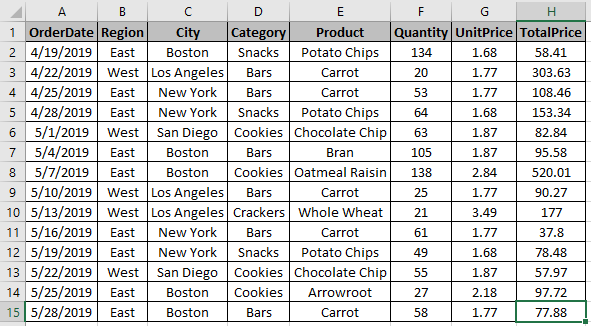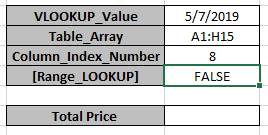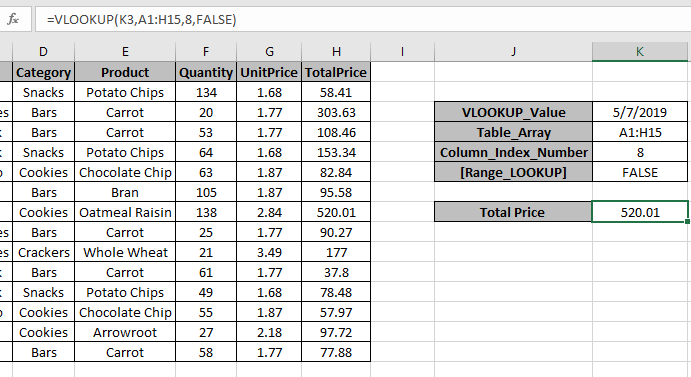# How to Vlookup by Date in Excel

In this article, we will learn about how to use VLOOKUP function in Excel to find the data by Date.

VLOOKUP just searches for the row of a given value in the first column of a table and returns asked column’s value in that row.
Syntax:

=VLOOKUP(lookup_date, table_array, col_index_number, [range_lookup])

Lookup_date: The date by which you want to search in the first column of Table Array.
Table_array: The Table in which you want to look up/search
col_index_number: The column number in Table Array from which you want to fetch results.
[range_lookup]: FALSE if you want to search for exact value, TRUE if you want an approximate Date match.

Here we know LOOKUP value is by Date
Let’s understand this function using it an example.
Here we have a Data Table and Vlookup Information and we need to find the Total Price by the date 5/7/2019.To find the value, use the Vlookup formula

=VLOOKUP(K3,A1:H15,8,FALSE)

Explanation:
The Formula looks up for the date in K3 cell in the table A1:H15.
Now once the date is found by the formula, It will lookup 8th column of the Table_array.
As the Last argument is False it will look for exact Date Match.As you can see here, the formula has the Total price for the provided date.

Hope you understood how to use VLOOKUP formula by Date in Excel. Explore more articles on Excel VLOOKUP function on Exceltip.com here. Please feel free to share your queries with us in the comment box below. We will help you.

Related Articles

Vlookup Top 5 Values with Duplicate Values Using INDEX-MATCH in Excel

How to VLOOKUP Multiple Values in Excel

How to VLOOKUP with Dynamic Col Index in Excel

How to Partial match with VLOOKUP function in Excel

How to use the VLOOKUP from Two or More Lookup Tables in Excel

How to use the Vlookup by Date in Excel

Using a VLOOKUP Formula to Check If a Value Exists

How to VLOOKUP from Different Excel Sheet in Excel

How to use the VLOOKUP with numbers and text in Excel

How to use the combination IF, ISNA and VLOOKUP function in Excel

How to use the combination of ISNA and VLOOKUP function in Excel

How to use the combination IFERROR and VLOOKUP function in Excel

Popular Articles:

50 Excel Shortcuts to Increase Your Productivity

How to use the VLOOKUP Function in Excel

How to use the COUNTIF function in Excel

How to use the SUMIF Function in Excel

Terms and Conditions of use

The applications/code on this site are distributed as is and without warranties or liability. In no event shall the owner of the copyrights, or the authors of the applications/code be liable for any loss of profit, any problems or any damage resulting from the use or evaluation of the applications/code.